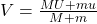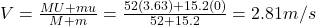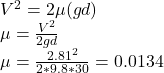## A 52.0-kg person, running horizontally with a velocity of +3.63 m/s, jumps onto a 15.2-kg sled that is initially at rest. (a) Ignoring the e

Question

A 52.0-kg person, running horizontally with a velocity of +3.63 m/s, jumps onto a 15.2-kg sled that is initially at rest. (a) Ignoring the effects of friction during the collision, find the velocity of the sled and person as they move away. (b) The sled and person coast 30.0 m on level snow before coming to rest. What is the coefficient of kinetic friction between the sled and the snow?

in progress 0
6 months 2021-07-21T12:53:40+00:00 1 Answers 28 views 0

The coefficient of kinetic friction between the sled and the snow is 0.0134

Explanation:

Given that:

M = mass of person = 52 kg

m = mass of sled = 15.2 kg

U = initial velocity of person = 3.63 m/s

u = initial velocity of sled = 0 m/s

After collision, the person and the sled would move with the same velocity V.

a) According to law of momentum conservation:

Total momentum before collision = Total momentum after collision

MU + mu = (M + m)VSubstituting values:The velocity of the sled and person as they move away is 2.81 m/s

b) acceleration due to gravity (g) = 9.8 m/s²

d = 30 m

Using the formula:The coefficient of kinetic friction between the sled and the snow is 0.0134